CBSE Sample Papers for Class 12 Physics Paper 6 are part of CBSE Sample Papers for Class 12 Physics. Here we have given CBSE Sample Papers for Class 12 Physics Paper 6.

## CBSE Sample Papers for Class 12 Physics Paper 6

 Board CBSE Class XII Subject Physics Sample Paper Set Paper 6 Category CBSE Sample Papers
Students who are going to appear for CBSE Class 12 Examinations are advised to practice the CBSE sample papers given here which is designed as per the latest Syllabus and marking scheme as prescribed by the CBSE is given here. Paper 6 of Solved CBSE Sample Paper for Class 12 Physics is given below with free PDF download solutions.
Time Allowed : 3 Hours
Max. Marks : 70
General Instructions
• All questions are compulsory. There are 26 questions in all.
• This question paper has five sections: Section A, Section B, Section C, Section D and Section E.
• Section A contains five questions of 1 mark each. Section B contains five questions of 2 marks each. Section C contains twelve questions of 3 marks each. Section D contains one value based question of 4 marks and Section E contains three questions of 5 marks each.
• There is no overall choice. However, an internal choice has been provided in 1 question of 2 marks, 1 question of 3 marks and all the 3 questions of 5 marks weightage. You have to attempt only 1 of the choices in such questions.
• You may use the following values of physical constants wherever necessary :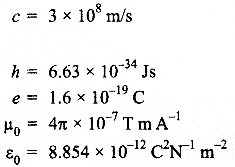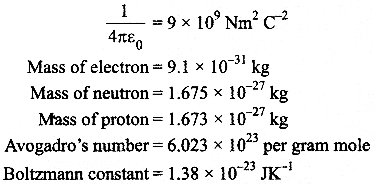Questions
SECTION : A

Question 1.
What are permanent magnets? Give one example.

Question 2.
What is the geometrical shape of equipotential surface due to a single isolated charge?

Question 3.
Which of the following waves can be polarized
(i) Heat waves

Question 4.
A capacitor has been charged by a dc source. What are the magnitude of conduction and displacement current, when it is fully charged?

Question 5.
Write the relationship between angle of incidence ‘i’, angle of prism ‘A’ and angle of minimum deviations Am for a triangular prism.

SECTION : B

Question 6.
The given graph shows the variation of photo-electric current (I) versus applied voltage (V) for two different photosensitive materials and for two different intensities of the incident radiations. Identify the pairs of curves that correspond to different materials but same intensity of incident radiation.

Question 7.
A 10 V battery of negligible internal resistance is connected across a 200 V battery and a resistance of 38 Ω as shown in the figure. Find the value of the current in circuit.

Question 8.
The emf of a cell is always greater than its terminal voltage. Why? Give reason.

Question 9.
(a) Write the necessary conditions for the phenomenon of total internal reflection to occur.
(b) Write the relation between the refractive index and critical angle for a given pair of optical media.

Question 10.
State Lenz’s law. A metallic rod held horizontally along east-west direction, is allowed to fall under gravity. Will there be an emf induced at its ends? Justify your answer.

SECTION : C

Question 11.
A convex lens of focal length 25 cm is placed co-axially in contact with a concave lens of focal length 20 cm. Determine the power of the combination. Will the system be converging or diverging in nature?

Question 12.
An ammeter of resistance 0.80 Ω can measure current up to 1.0 A.
(i) What must be the value of shunt resistance to enable the ammeter to measure current up to 5.0 A?
(ii) What is the combined resistance of the ammeter and the shunt?

Question 13.
In the given circuit diagram a voltmeter ‘V is connected across a lamp ‘L’. How would
(i) the brightness of the lamp and
(ii) voltmeter reading ‘V’ be affected, if the value of resistance ‘R’ is decreased? Justify your answer.

Question 14.
(a) An EM wave is travelling in a medium with a velocity v = v$$\overrightarrow { i }$$ . Draw a sketch showing the propagation of the EM wave, indicating the direction of the oscillating electric and magnetic fields.
(b) How are the magnitudes of the electric and magnetic fields related to velocity of the EM wave?

Question 15.
Block diagram of a receiver is shown in the figure :
(a) Identify ‘X’ and ‘Y’
(b) Write their functions.Question 16.
Explain, with the help of a circuit diagram, the working of a photo diode. Write briefly how it is used to detect the optical signals.

OR

Mention the important consideration required while fabricating a pn-j unction diode to be used as a Light Emitting Diode (LED). What should be the order of band gap of an LED if it is required to emit light in the visible range?

Question 17.
(a) Why photoelectric effect cannot be explained on the basis of wave nature of light? Give reasons.
(b) Write the basic features of photon picture of electromagnetic radiation on which Einstein’s photoelectric equation is based.

Question 18.
A capacitor of unknown capacitance is connected across a battery of V volts. The charge stored in it is 360 μC. When potential across the capacitor is reduced by 120 V, the charge stored in it becomes 120 μC.
Calculate :
(i) The potential V and the unknown capacitance C.
(ii) What will be the charge stored in the capacitor, if the voltage applied had increased by 120 V?

OR

A hollow cylindrical box of length 1 m and area of cross section 25 cm is placed in a three dimensional coordinate system as shown in the figure. The electric field in the region is given by $$\overrightarrow { E }$$ = 5Ox $$\overrightarrow { i }$$ where E is in NC andx is in metres.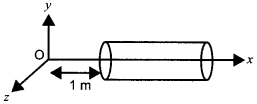Find
(i) Net flux through the cylinder.
(ii) Charge enclosed by the cylinder.

Question 19.
(a) In a typical nuclear reaction, e.g.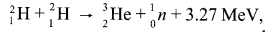although number of nucleons is conserved, yet energy is released. How? Explain.
(b) Show that nuclear density in a given nucleus is independent of mass number A.

Question 20.
Write three important factors which justify the need of modulating a message signal. Show diagrammatically how an amplitude modulated wave is obtained when a modulating signal is superimposed on a carrier wave.

Question 21.
A metallic rod of length L is rotated with a frequency v with one end hinged at the centre and the other end at the circumference of a circular metallic ring of radius r, about an axis passing through the centre and perpendicular to the plane of the ring. A constant uniform magnetic field B parallel to the axis is present everywhere. Using Lorentz force, explain how emf is induced between the centre and the metallic ring and hence obtained the expression for it.

Question 22.
Output characteristics of an n-p-n transistor in CE configuration is shown in the figure.
Determine :
(i)
dynamic output resistance
(ii) dc current gain and
(iii) ac current gain at an operating point VCE = 10 V, when IB = 30 pA.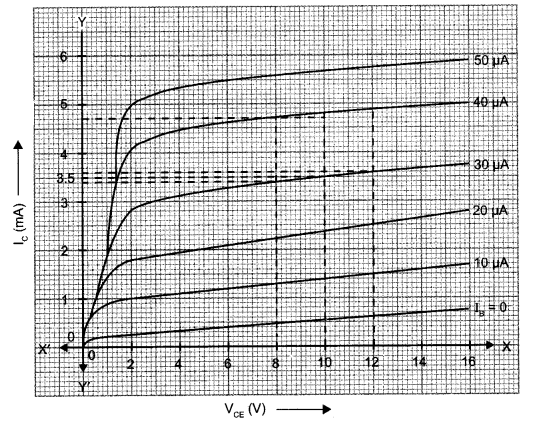SECTION : D

Question 23.
While travelling back to his residence in the car, Mr. Ram was caught up in a thunderstorm. He stopped driving the car and waited for thunderstorm to stop. He saw a child standing under the tree. He asked the boy not to stand under a tree in thunderstorm and to come inside the car till the thunderstorm stopped. Later he dropped the boy at his residence. The boy requested that he should meet his parents. The parents expressed their gratitude to him for his concern for safety of their child.
Answer the following questions based on the above information :

1. Why is it safer to sit inside a car during a thunderstorm?
2. Which two values are displayed by Mr. Ram in his action?
3. Which values are reflected in parents’ response to Mr. Ram?
4. Give an example of similar action on your part from everyday life.

SECTION : E

Question 24.
(a) Draw a ray diagram showing the image formation by a compound microscope. Hence obtain expression for total magnification when the image is formed at infinity.

OR

(a) State Huygens principle. Using this principle draw a diagram to show how a plane wave front incident at the interface of the two media gets refracted when it propagates from a rarer to a denser medium. Hence verify Snell’s law of refraction.
(b) When monochromatic light travels from a rarer to a denser medium, explain the following, giving reasons:
(i) Is the frequency of reflected and refracted light same as the frequency of incident light?
(ii) Does the decrease in speed imply a reduction in the energy carried by light wave?

Question 25.
(a) State the working principle of a potentiometer. With the help of the circuit diagram, explain how a potentiometer is used to compare the emfs of two primary cells.  Obtain the required expression used for comparing the emfs.
(b) Write two possible causes for one sided deflection in a potentiometer experiment.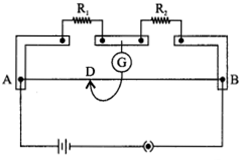OR

(a) State Kirchhoff’s rules for an electric network. Using Kirchhoff’s rules, obtain the balance condition in terms of the resistance of four arms of Wheatstone bridge.
(b) In the meter bridge experimental set up, shown in the figure, the null point ‘D’ is obtained at a distance of 40 cm from end A of the meter bridge wire. If a resistance of 10 Q is connected in series with R1, null point is obtained at AD = 60 cm. Calculate the values of R1 and R2

Question 26.
(a) Derive the expression for the torque on a rectangular current carrying loop suspended in a uniform magnetic field.
(b) A proton and a deuteron having equal momenta enter in a region of a uniform magnetic field at right angle to the direction of the field. Depict their trajectories in the field.

OR

(a) A small compass needle of magnetic moment ‘m’ is free to tum about an axis perpendicular to the direction of uniform magnetic field ‘B’. The moment of inertia of the needle about the axis is T. The needle is slightly disturbed from its stable position and then released. Prove that it executes simple harmonic motion. Hence deduce the expression for its time period.
(b) A compass needle, free to turn in a vertical plane orients itself with its axis vertical at a certain place on the earth. Find out the values of
(i) horizontal component of earth’s magnetic field and
(ii) angle of dip at the place.

SECTION : A

The magnets which have high retentivity and high coercivity are known as permanent magnets. For example : Steel.

The equipotential surfaces of an isolated charge are concentric spherical shells and the distance between the shells increase with the decrease in electric field and vice-versa.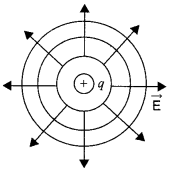Heat waves can be polarized as they are transverse waves whereas sound waves cannot be polarized as they are longitudinal waves. Transverse waves can oscillate in the direction perpendicular to the direction of its transmission but longitudinal waves oscillate only along the direction of its transmission. So, longitudinal waves cannot be polarized.

Electric flux through plates of capacitor,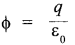Here q is constant, the capacitor is fully charged.As voltage becomes constant when capacitor is fully charged.

The relation between the angle of incidence i, angle of prism A, and the angle of minimum deviation A , for a triangular prism is given by i = (A + Am)/2

SECTION : B

Curves 1 and 2 correspond to similar materials while curves 3 and 4 represent different materials, since the value of stopping potential for 1,2 and 3,4 are the same. For the given frequency of the incident radiation, the stopping potential is independent of its intensity.So, the pairs of curves ( 1 and 3) and (2 and 4) correspond to different materials but same intensity of incident radiation.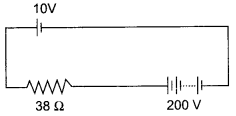Since, the positive terminal of the batteries are connected together, so the equivalent emf of the batteries is given by
E = 200- 10= 190 V
Hence, the current in the circuit is given by
I = E/R = 190/38 = 5 A

The emf of a cell is greater than its terminal voltage because there is some potential drop across the cell due to its small internal resistance.

(a) Necessary conditions for total internal reflection to occur are :

1. The incident ray on the interface should travel in optically denser medium.
2. The angle of incidence should be greater than the critical angle for the given pair of optical media.

(b)Where a and b are the rarer and denser media respectively. C is the critical angle for the given pair of optical media.

Lenz’s law states that the polarity of induced emf is such that it produces a current which opposes the change in magnetic flux that produces it. Emf will be induced in the rod as there is change in magnetic flux.

When a metallic rod held horizontally along east-west direction, is allowed to fall freely under gravity i.e. fall from north to south, the magnetic flux changes and the emf is induced in it.

SECTION : C

We have focal length of convex lens,
f1  = +25 cm = +0.25 m
Focal length of the concave lens,
f1 = -20 cm = -0.20 m
Equivalent focal length,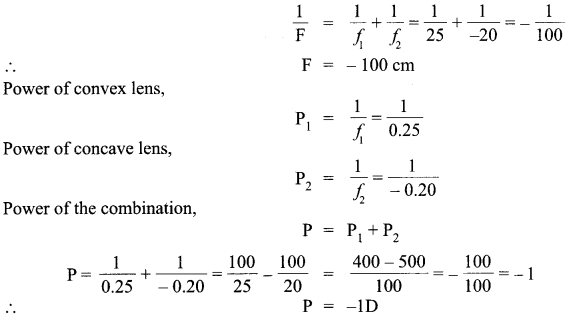∴ The focal length of the combination = -1 m = -100 cm As the focal length is negative, the system will be diverging in nature.

We have, resistance of ammeter, RA = 0.80 ohm
Maximum current across ammeter, IA = 1.0 A.
So, voltage across ammeter, V= IR = 1 x 0.80 = 0.8 V
Let the value of shunt be x.
(i) Resistance of ammeter with shunt,(ii) Combined resistance of the ammer and the shunt,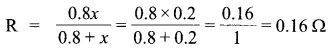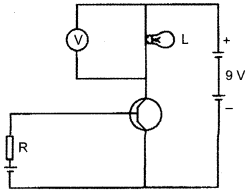The given figure is Common Emitter (CE) configuration of an n-p-n transistor. The input circuit is forward biased and collector circuit is reverse biased. If resistance R decreases, forward biased in the input circuit will increase, thus the base current (IB) will decrease and the emitter current (IE) will increase. This will increase the collector current (Ic) as
(IE) = (IB) + (Ic).
When Ic increases which flows through the lamp, the voltage across the bulb will also increase making the lamp brighter and the voltmeter is connected in parallel with the lamp, the reading in the voltmeter will also increase.

(a) Given,
Velocity, v = v $$\overrightarrow { i }$$ and E is the electric field along Y-axis and B is the magnetic field along Z-axis.
The propagation of EM wave is shown below :(b) Speed of EM wave can be given as the ratio of magnitude of electric field (E0) to the magnitude of magnetic field (B0)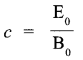From the given block diagram of demodulator of a typical receiver, we can conclude the following things :
(a) X represents Intermediate Frequency (IF) stage while Y , represents an amplifier.
(b) At IF stage, the carrier frequency is transformed to a lower frequency then in this process, the modulated signal is detected. The function of amplifier is to amplify the detected signal which may not be strong enough to be made use of and hence is essential.

A junction diode made from light sensitive semiconductor is called a photo diode.An electrical device that is used to detect and convert light into an energy signal with the use of a photo detector is known as a photo diode. The light that falls on it controls the function of pn-junction. Suppose, the wavelength is such that the energy of a photon hcfk is enough to break a valence bond. There is an increase in number of charge carriers and hence the conductivity of the junction also increases. New hole-electron pairs are created when such light falls on the junction. If the junction is connected in a circuit, the intensity of the incident light controls the current in the circuit.

OR

1. The reverse breakdown voltage of LEDs are very low, which is around 5 V. So enough care is to be taken while fabricating a pn-junction diode such that the high reverse voltages do not occur across them.
2. There exist very small resistance to limit the current in LED. So, a resistor must be placed in series with the LED such that no damage is occurred to the LED.The semiconductor used for fabrication of visible LEDs must atleast have a band gap of 1.8 eV.

(a) Wave nature of radiation cannot explain the photoelectric effect because of :

1. The immediate ejection of photo electrons.
2. The presence of threshold frequency for a metal surface.
3. The fact that kinetic energy of the emitted electrons is independent of the intensity of light and depends upon its frequency. Thus, the photoelectric effect cannot be explained on the basis of wave nature of light.

(b) Photon picture of electromagnetic radiation on which Einstein’s photoelectric equation is based on particle nature of light. Its basic features are :

1. In interaction with matter, radiation behaves as if it is made up of particles called photons.
2. Each photon has energy (E = hv), momentum (p =hv /c) , and speed c, the speed of light.
3. All photons of light of a particular frequency v, or wavelength X, have the same energy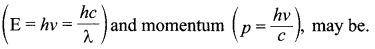4. By increasing the intensity of light of given wavelength, there is only an increase in the number of photons per second crossing a given area, with each photon having the same energy. Thus, photon energy is independent of intensity’ of radiation.
5. Photons are electrically neutral and are not deflected by electric and magnetic fields.
6. In a photon – particle collision (such as photon-electron collision), the total energy and total momentum are conserved. However, number of photons may not be conserved.

(i) Initial voltage, V1, = V volts and charge stored, Q1 = 360 μC
Q1 = CV1            ………(1)
Changed potential,
V2 = V -120
Q2 = 120 μC
Q2 = CV2       ………. (2)
By dividing (1) by (2), we get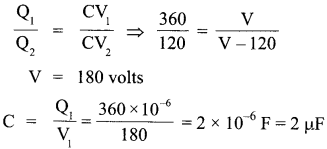(ii) If the voltage applied had increased by 120 V, then
V3 =180 + 120 = 300 V
Hence, charge stored in the capacitor,
Q3 = CV3  = 2 x 10-6  x300 = 600 μC

OR

(i) Given,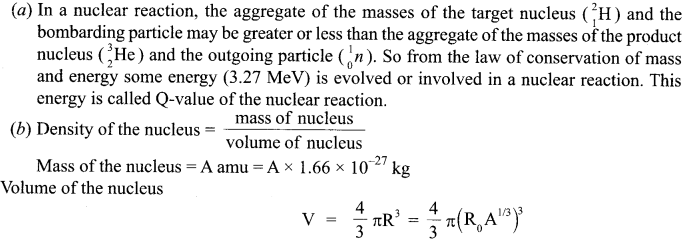Which shows that the density is independent of mass number A.

Three important factors which justify the need of modulating a message signal :(i) Size of antenna or aerial :
For communication within the effective length of the antennas, the transmitting frequencies should be high, so modulation is required.
(ii) Effective power which is radiated by antenna :
Since the power radiated from a linear antenna is inversely proportional to the square of the transmitting wavelength. As high powers are needed for good transmission, so higher frequency is required which can be achieved by modulation.
(iii) The interference of signals from different transmitters :
To avoid the interference of the signals there is a need of high frequency which can be achieved by the modulation.

Suppose the length of the rod is greater than the radius of the circle and rod rotates anticlockwise and suppose the direction of electrons in the rod at any instant be along +y direction. Suppose the direction of the magnetic field is along +z direction.
Then, using Lorentz law, we get the following :Thus, the direction of force on the electrons is along x-axis. So, the electrons will move towards the centre i.e., the fixed end of the rod. This movement of electrons will result in current and thus it will generate an emf in the rod between the fixed end and the point touching the ring.
Let 0 be the angle between the rod and radius of the circle at any time t.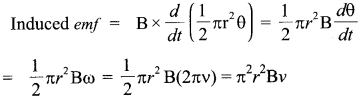(i) Dynamic output resistance is given as :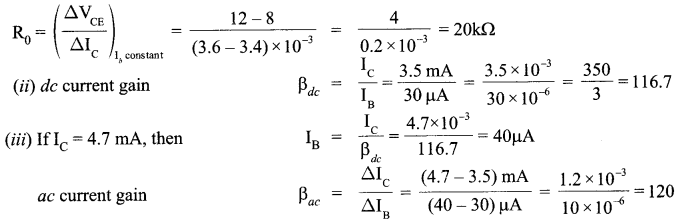SECTION : D

1. It is safer to be inside a car during thunderstorm because the car acts like a Faraday cage.
2. Awareness and humanity
3. Gratitude and obliged
4. Once I came across to a situation where a puppy was shuck in the middle of a busy road during rain and was not able to cross due to heavy flow, so I quickly rushed and helped him.

SECTION : E

(a) A compound microscope consists of two convex lenses parallel separated by some distance. The lens nearer to the object is called the objective. The lens through which the final image is viewed is called the eyepiece. Magnifying power, when final image is at infinity: The magnification produced by the compound microscope is the product of the magnifications produced by the eyepiece and objective.
M = Me x M0       ……… (i)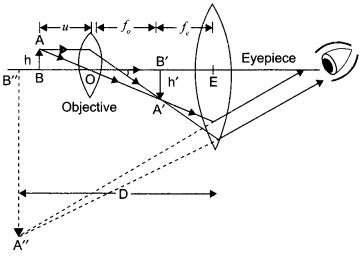∴ Where, Me and M0 are the magnifying powers of the eyepiece and objective respectively.
If u() is the distance of the object from the objective and v0 is the distance of the image from the objective, then the magnifying power of the objective isWhere, h, h’ are object and image heights ; respectively and f0 is the focal length of the a” objective.
L is the tube length i.e., the distance between the second focal point of the objective and the first focal point of the eyepiece : When the final image is at infinity,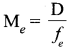Magnifying power of compound microscope,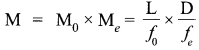OR

(a) Huygens Principle :

• Each point on the primary wavefront acts as a source of secondary wavelets, transferring out disturbance in all directions in the same way as the original source of light does.
• The new position of the wavefront at any instant is the envelope of the secondary wavelets at that instant.Refraction on the basis of wave theory
• Consider any point Q on the incident wave front.
• Suppose when disturbance from point P on incident wave front reaches point P’ on the refracted wavefront, the disturbance from point Q reaches Q’ on the refracting surface XY.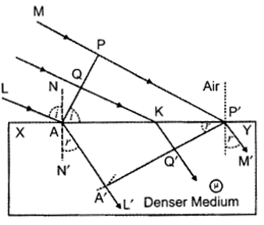Since P’A’ represents the refracted wavefront, the time taken by light to travel from a point on incident wavefront to the corresponding point on refracted wavefront should always be the same. Now, time taken by light to go from Q to Q’ will be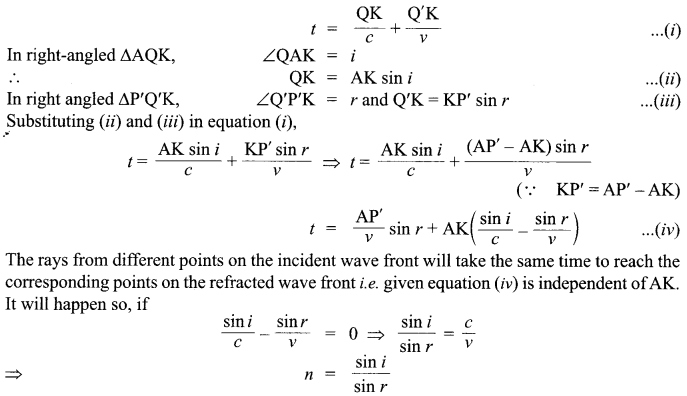This is the Snell’s law for refraction of light.

(b)

1. The frequency of reflected and refracted light remains constant as the frequency of incident light only depends on the source of light.
2. Since the frequency remains same, hence there is no reduction in energy.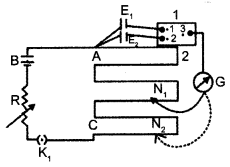(a) Working principle of Potentiometer :
When a constant current is passed through a wire of uniform area of cross-section, the potential drop across any portion of the wire is directly proportional to the length of that portion.

Applications of Potentiometerfor comparing emfs of two cells :
The following figure shows an application of the potentiometer to compare the emf of two cells of emf Eand E,. 1, 2, 3 form a two way key.
When 1 and 3 are connected, E1 is connected to the galvanometer (G). Jockey is moved to N1 which is at a distance l1 from A, to find the balancing length. Applying loop rule to AN1G31 A,Thus, we can compare the emfs of any two sources. Generally, one of the cells is chosen as a standard cell whose emf is known to a high degree of accuracy. The emf of the other cell is then calculated from equation (3).

(b)

1. The emf of the cell connected in main circuit may not be more than the emf of the primary cells whose emfs are to be compared.
2. The positive ends of all cells are not connected to the same end of the wire.

OR

(a) Kirchhoff’s First Law – Junction Rule :
The algebraic sum of the currents meeting at a point in an electrical circuit is always zero.
Let the currents be I1  , I2 , I3 and I4Convention :
Current towards the junction – positive Current away from the junction – negative
I3+ (- I1) + (- I2) +(- I4) = 0

Kirchhoff’s Second Law – Loop Rule :
In a closed loop, the algebraic sum of the emfs is equal to the algebraic sum of the products of the resistance and current flowing through them.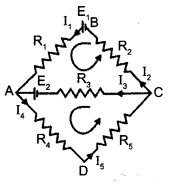For closed part BACB,
E+ E2  =  I1 R1– I3 R3  + I2 R2
E2 =  I3 R  + I4 R  + I5 R5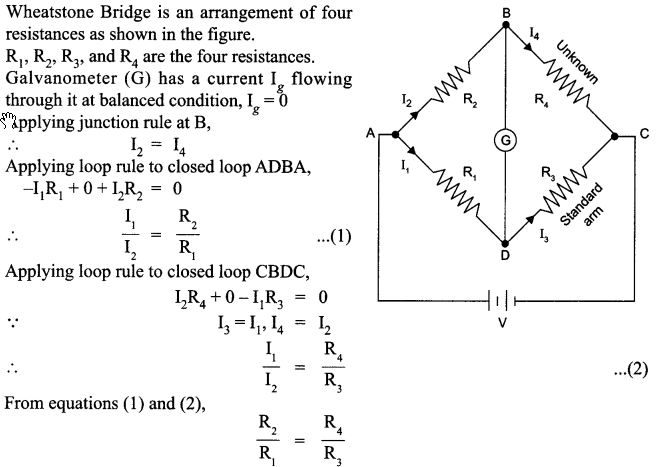(b) Considering both the situations and writing them in the form of equations. Let R’ be the resistance per unit lenght of the potentiometer wire,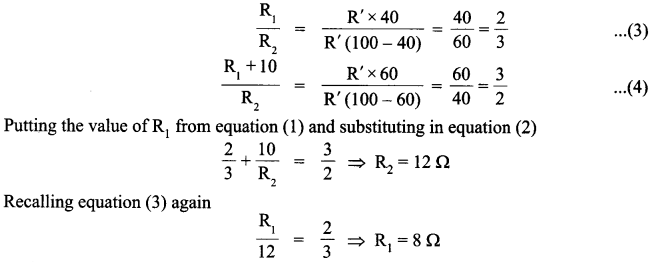(a) Consider a rectangular loop-ABCD carrying current I.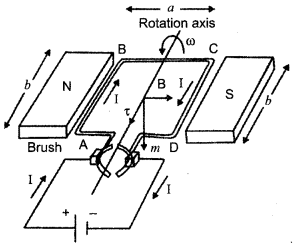Case I :
The rectangular loop is placed such that the uniform magnetic field B is in the plane of loop. No force is exerted by the magnetic field on the arms AD and BC. Magnetic field exerts a force
∴  F1 = IbB  = F1
Magnetic field exerts a force F2 on arm CD.
F2 = IbB = F1Net force on the loop is zero.
The torque on the loop rotates the loop in anti-clockwise direction.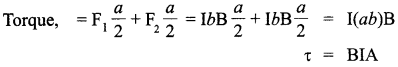τ = BIA
If there are n such turns the torque will be nIAB
Where, b →Breadth of the rectangular coil
a → Length of the rectangular coil
A = ab → Area of the coil.

Case II :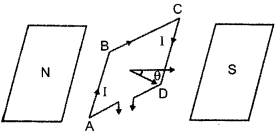Plane of the loop is not along the magnetic field, but makes angle with it. Angle between the field and the normal is θ. Forces on BC and DA are equal and opposite and they cancel each other as they are collinear. Force on AB is F, and force on CD is F2.
F1= F2 = IbBWhere A = ab
(b) We know, Lorentz force, F = Bqv sin θ
Where θ = angle between velocity of particle and magnetic field = 90
So, Lorentz force, F = Bqv
Thus, the particles will move in circular path.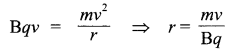Let mp = mass of proton, md = mass of deuteron, v = velocity of proton and vd = velocity of deuteron The charge of proton and deuteron are equal.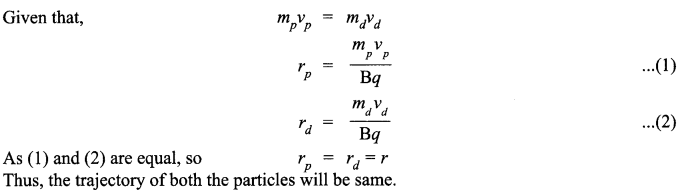As (1) and (2) are equal, so Thus, the trajectory of both the particles will be same.

OR

(a) The torque on the needle is τ = m x B In magnitude, τ= mB sin θ
Here τ is restoring torque and 0 is the angle between m and B.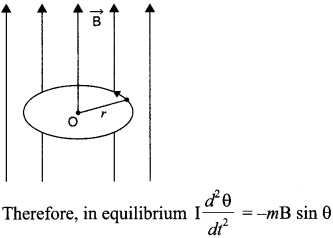Negative sign with mB sin θ implies that restoring torque is in opposition to deflecting torque. For small values of 0 in radians, we approximate sin θ = θ and get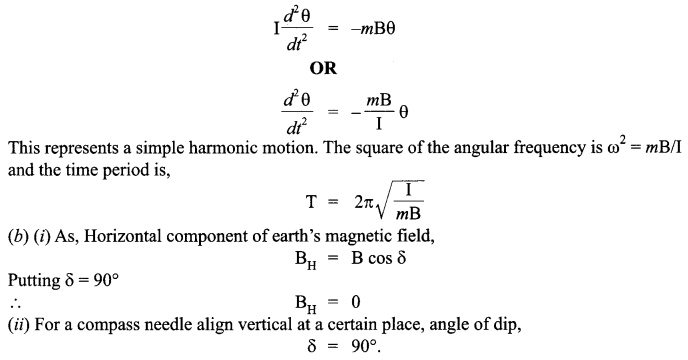We hope the CBSE Sample Papers for Class 12 Physics Paper 6 help you. If you have any query regarding CBSE Sample Papers for Class 12 Physics Paper 6, drop a comment below and we will get back to you at the earliest.